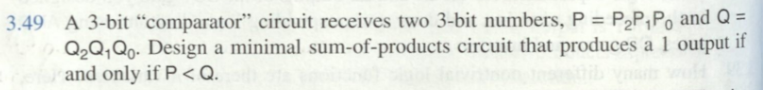# Question Design a circuit 3.49 A 3-bit "comparator" circuit receives two 3-bit numbers, $$P=P_{2} P_{1} P_{0}$$ and $$Q=$$ $$Q_{2} Q_{1} Q_{0}$$. Design a minimal sum-of-products circuit that produces a 1 output if and only if $$\mathrm{P}<\mathrm{Q}$$.U5WTSC The Asker · Electrical Engineering

Design a circuitTranscribed Image Text: 3.49 A 3-bit "comparator" circuit receives two 3-bit numbers, $$P=P_{2} P_{1} P_{0}$$ and $$Q=$$ $$Q_{2} Q_{1} Q_{0}$$. Design a minimal sum-of-products circuit that produces a 1 output if and only if $$\mathrm{P}<\mathrm{Q}$$.
More
Transcribed Image Text: 3.49 A 3-bit "comparator" circuit receives two 3-bit numbers, $$P=P_{2} P_{1} P_{0}$$ and $$Q=$$ $$Q_{2} Q_{1} Q_{0}$$. Design a minimal sum-of-products circuit that produces a 1 output if and only if $$\mathrm{P}<\mathrm{Q}$$.